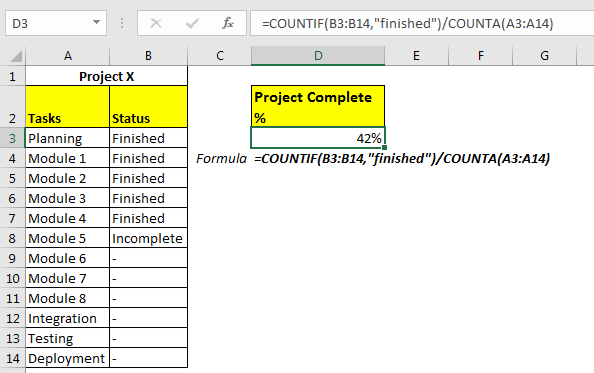# How to Calculate Project Complete Percentage in Excel

A project is often divided into smaller tasks. When each task is completed, we say that project is completed 100%. In that case we would  like top monitor how task is completed while we work on the project. In this article, we will learn how you can calculate the project complete percentage in Excel

Generic Formula For Project Complete %

Status range : It is the range in which status of task is written.

Finished: Here “finished” word is used when a task of project is completed. You can use any word you want.

Let’s see an example to make things clear.
Example: Calculate Project Complete Percentage in ExcelHere I have a list of tasks in range A3:A14. Their status is written in range B3:B14.
We need to calculate percent complete in excel.

Apply above generic percent complete formula

=COUNTIF(B3:B14,"finished")/COUNTA(A3:A14)

It will return a fraction number. Change the formate to percentage using excel shortcut CTRL+SHIFT+5. And it’s done.How it works?

Well, it is a simple excel formula. We count the number of completed tasks in excel using COUNTIF(B13:B14, “finished”).

Than we divided the number of completed tasks from total number of tasks using /COUNTA(A3:A14).
Finally we formated that cell as percentage. And that is it. We have calculated percentage of project completion in excel using a simple formula.
So yeah guys, this how you calculate total percentage in excel. Let me know if you have any doubts regarding this excel topic or any other. The comments section is all yours.

Related Articles

Calculate Percentage of Total in Excel

Calculate Percentage Discount

Increase by percentage

Calculate Profit margin percentage

Popular Articles:

The VLOOKUP Function in Excel

COUNTIF in Excel 2016

How to Use SUMIF Function in Excel

Terms and Conditions of use

The applications/code on this site are distributed as is and without warranties or liability. In no event shall the owner of the copyrights, or the authors of the applications/code be liable for any loss of profit, any problems or any damage resulting from the use or evaluation of the applications/code.